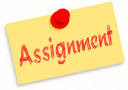###Programs Assignment – 2018

1. Print 'hello world' in java.
2. Addition of two values passing by command line argument.
3. Write a program to find the result of following expression.
1) a << 2 + b >> 2
2) (a<<2) + (b>>2)
3) a & b
4) a | 4 + a >> b & 7
4. Write a program in java to explain the use of break and continue statements.
5. Create Box class with data members width, height and depth. Objects of this class initializewith zero value or by passing single value. Create a method to calculate volume and display it.
6. Calculate area of square, rectangle and triangle using the three same name methods 'area';
7. Make CALC class with two data members. Perform addition, subtraction, multiplication and division operation of given two values by user. Initialize both data members with zero. (use menu-driven)
8. Create array with values {8,7,6,5,4} and display it.